【為什麼我們要挑選這篇文章】神經網路是目前人工智慧最常使用的一種模型，用tensorflow、pytorch 寫出神經網路並不稀奇，但，該怎麼只用 python 和 numpy 搭建出神經網路？最近有神人寫了手把手教學文，教你用 100 行 python，輕鬆搞定神經網路。（責任編輯：藍立晴）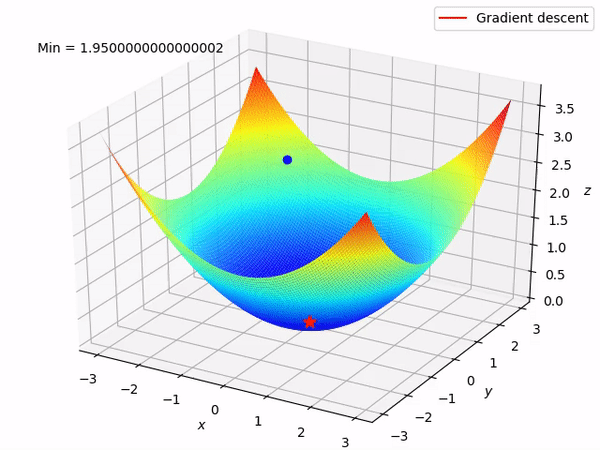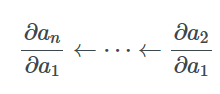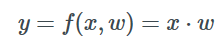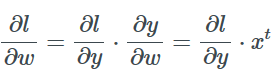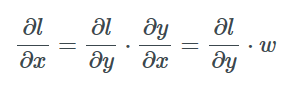``````class Layer:
def __init__(self):
self.parameters = []

def forward(self, X):
"""
Override me! A simple no-op layer, it passes forward the inputs
"""
return X, lambda D: D

def build_param(self, tensor):
"""
Creates a parameter from a tensor, and saves a reference for the update step
"""
param = Parameter(tensor)
self.parameters.append(param)
return param

def update(self, optimizer):
for param in self.parameters: optimizer.update(param)``````

``````class SGDOptimizer():
def __init__(self, lr=0.1):
self.lr = lr

def update(self, param):

``````class Linear(Layer):
def __init__(self, inputs, outputs):
super().__init__()
tensor = np.random.randn(inputs, outputs) * np.sqrt(1 / inputs)
self.weights = self.build_param(tensor)
self.bias = self.build_param(np.zeros(outputs))

def forward(self, X):
def backward(D):
return D @ self.weights.tensor.T
return X @ self.weights.tensor +  self.bias.tensor, backward``````

``````class ReLu(Layer):
def forward(self, X):

``````class Sigmoid(Layer):
def forward(self, X):
S = 1 / (1 + np.exp(-X))
def backward(D):
return D * S * (1 - S)
return S, backward``````

``````class Sequential(Layer):
def __init__(self, *layers):
super().__init__()
self.layers = layers
for layer in layers:
self.parameters.extend(layer.parameters)

def forward(self, X):
backprops = []
Y = X
for layer in self.layers:
Y, backprop = layer.forward(Y)
backprops.append(backprop)
def backward(D):
for backprop in reversed(backprops):
D = backprop(D)
return D
return Y, backward``````

``````def mse_loss(Yp, Yt):
diff = Yp - Yt
return np.square(diff).mean(), 2 * diff / len(diff)``````

``````class Learner():
def __init__(self, model, loss, optimizer):
self.model = model
self.loss = loss
self.optimizer = optimizer

def fit_batch(self, X, Y):
Y_, backward = self.model.forward(X)
L, D = self.loss(Y_, Y)
backward(D)
self.model.update(self.optimizer)
return L

def fit(self, X, Y, epochs, bs):
losses = []
for epoch in range(epochs):
p = np.random.permutation(len(X))
X, Y = X[p], Y[p]
loss = 0.0
for i in range(0, len(X), bs):
loss += self.fit_batch(X[i:i + bs], Y[i:i + bs])
losses.append(loss)
return losses``````

``````X = np.random.randn(100, 10)
w = np.random.randn(10, 1)
b = np.random.randn(1)
Y = X @ W + B

model = Linear(10, 1)
learner = Learner(model, mse_loss, SGDOptimizer(lr=0.05))
learner.fit(X, Y, epochs=10, bs=10)``````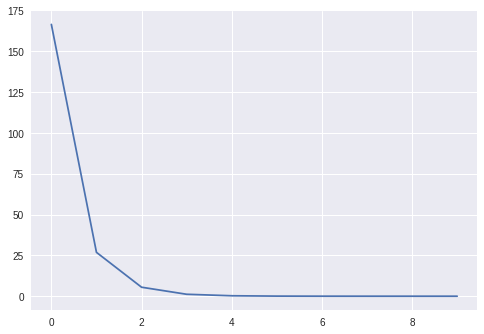``print(np.linalg.norm(m.weights.tensor - W), (m.bias.tensor - B))``> 1.848553648022619e-05 5.69305886743976e-06``

``````X = np.random.randn(1000, 2)
Y = X[:, 0] * X[:, 1]

losses1 = Learner(
Sequential(Linear(2, 1)),
mse_loss,
SGDOptimizer(lr=0.01)
).fit(X, Y, epochs=50, bs=50)

losses2 = Learner(
Sequential(
Linear(2, 10),
Sigmoid(),
Linear(10, 1)
),
mse_loss,
SGDOptimizer(lr=0.3)
).fit(X, Y, epochs=50, bs=50)

plt.plot(losses1)
plt.plot(losses2)
plt.legend(['1 Layer', '2 Layers'])
plt.show()``````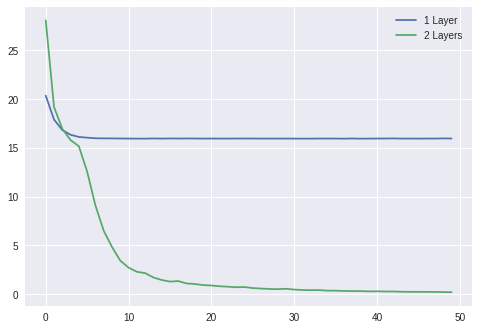（本文經 大數據文摘 授權轉載，並同意 TechOrange 編寫導讀與修訂標題，原文標題為 〈100行Python代码，轻松搞定神经网络〉 。首圖來源：Flickr，CC Licensed）